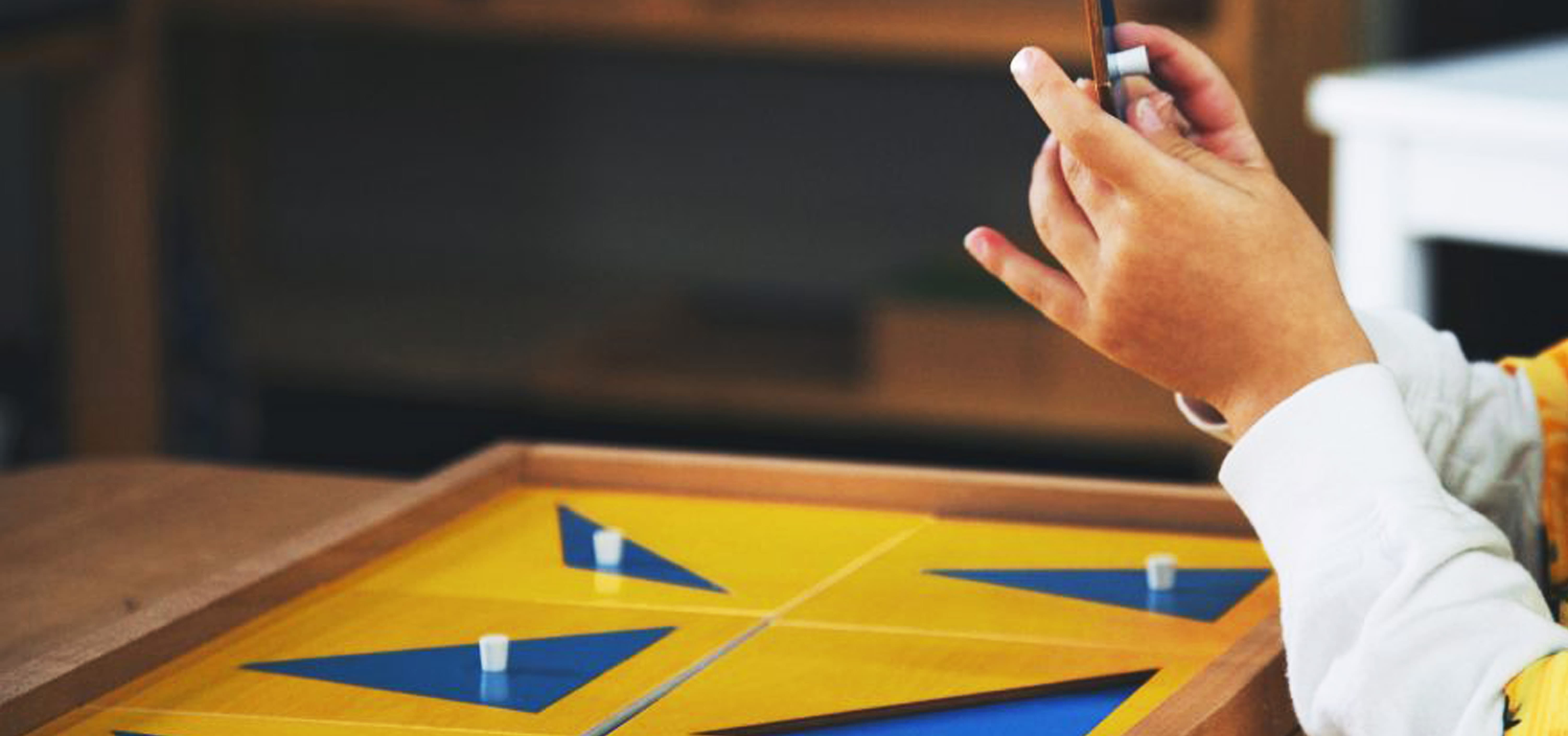Nirmala V Mani

## Montessori Materials Spotlight - Geometric Cabinet - Triangles

The Geometric Cabinet is a key component of Montessori education that helps children to develop an understanding of basic geometric shapes. It consists of a set of wooden trays with different geometric shapes, including triangles, circles, squares, and polygons. In this blog, we will focus on the triangle tray and explore the different types of triangles, including equilateral, isosceles, and scalene triangles, and how they can be taught using the Geometric Cabinet.

### Main types of triangles

• An equilateral triangle is a triangle in which all three sides are equal in length. It is also an example of a regular polygon because all angles and sides are the same.
• An isosceles triangle is a triangle in which two sides are of equal length, and the third side is of a different length. This type of triangle has two equal angles opposite the equal sides.
• A scalene triangle is a triangle in which all three sides are of different lengths. It does not have any equal angles or sides.### Teaching Triangles with the Geometric Cabinet

The triangle tray in the Geometric Cabinet includes a set of equilateral, isosceles, and scalene triangles in various sizes and colours. Children are introduced to the triangle tray after they have mastered the square tray and have developed an understanding of basic shapes and sizes.

The teacher introduces the different types of triangles to the children and explains their characteristics, including the number of sides and angles, as well as their unique properties. The children are then invited to explore the triangle tray and match the triangles with the corresponding insets on a separate board. This activity helps children to develop their visual discrimination skills and refine their ability to identify and classify different types of triangles.

After the children have become familiar with the different types of triangles, the teacher can introduce more complex activities that involve creating geometric designs using the triangles. For example, the children can be asked to create a large equilateral triangle using smaller equilateral triangles or to create a pattern using isosceles triangles of different sizes and colours.

Thus, the Geometric Cabinet is an essential tool in Montessori education that helps children to develop an understanding of basic geometric shapes, including triangles. Moreover, by incorporating geometric activities into the curriculum, we can help to promote a love for learning and a deeper understanding of the world around us.